• 优化器总结 机器学习中，有很多优化方法来试图寻找模型的最优解。比如神经网络中可以采取最基本的梯度下降法。 梯度下降法(Gradient Descent) 梯度下降法是最基本的一类优化器，目前主要分为三种梯度下降法：...
优化器总结
机器学习中，有很多优化方法来试图寻找模型的最优解。比如神经网络中可以采取最基本的梯度下降法。

1. 标准梯度下降法(GD)
假设要学习训练的模型参数为

W

W

$W$，代价函数为J(W)$J\left(W\right)$$J(W)$，则代价函数关于模型参数的偏导数即相关梯度为

ΔJ(W)

Δ

J

(

W

)

$\Delta J(W)$，学习率为

ηt

η

t

$\eta_t$，则使用梯度下降法更新参数为：

Wt+1=Wt−ηtΔJ(Wt)

W

t

+

1

=

W

t

−

η

t

Δ

J

(

W

t

)

W_{t+1} = W_t - \eta_t \Delta
J(W_t)其中，

Wt

W

t

$W_t$表示

t

t

$t$时刻的模型参数。
从表达式来看，模型参数的更新调整，与代价函数关于模型参数的梯度有关，即沿着梯度的方向不断减小模型参数，从而最小化代价函数。
基本策略可以理解为”在有限视距内寻找最快路径下山“，因此每走一步，参考当前位置最陡的方向(即梯度)进而迈出下一步。可以形象的表示为：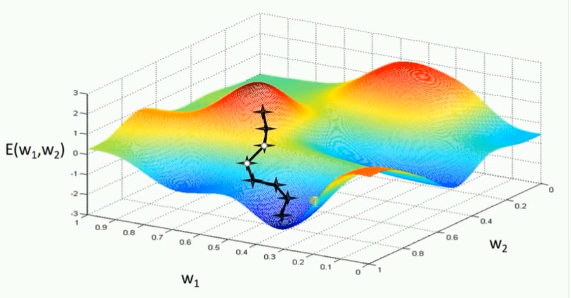评价：标准梯度下降法主要有两个缺点:

训练速度慢：每走一步都要要计算调整下一步的方向，下山的速度变慢。在应用于大型数据集中，每输入一个样本都要更新一次参数，且每次迭代都要遍历所有的样本。会使得训练过程及其缓慢，需要花费很长时间才能得到收敛解。
容易陷入局部最优解：由于是在有限视距内寻找下山的反向。当陷入平坦的洼地，会误以为到达了山地的最低点，从而不会继续往下走。所谓的局部最优解就是鞍点。落入鞍点，梯度为0，使得模型参数不在继续更新。

2. 批量梯度下降法(BGD)

假设批量训练样本总数为n$n$$n$，每次输入和输出的样本分别为

X(i),Y(i)

X

(

i

)

,

Y

(

i

)

$X^{(i)}, Y^{(i)}$，模型参数为

W

W

$W$，代价函数为J(W)$J\left(W\right)$$J(W)$，每输入一个样本

i

i

$i$代价函数关于W$W$$W$的梯度为

ΔJi(Wt,X(i),Y(i))

Δ

J

i

(

W

t

,

X

(

i

)

,

Y

(

i

)

)

$\Delta J_i(W_t, X^{(i)}, Y^{(i)})$，学习率为

ηt

η

t

$\eta_t$，则使用批量梯度下降法更新参数表达式为：

Wt+1=Wt−ηt∑i=1nΔJi(Wt,X(i),Y(i))

W

t

+

1

=

W

t

−

η

t

∑

i

=

1

n

Δ

J

i

(

W

t

,

X

(

i

)

,

Y

(

i

)

)

W_{t+1} = W_t - \eta_t \sum_{i=1}^n \Delta J_i(W_t, X^{(i)}, Y^{(i)})其中，

Wt

W

t

$W_t$表示

t

t

$t$时刻的模型参数。
从表达式来看，模型参数的调整更新与全部输入样本的代价函数的和（即批量/全局误差）有关。即每次权值调整发生在批量样本输入之后，而不是每输入一个样本就更新一次模型参数。这样就会大大加快训练速度。
基本策略可以理解为，在下山之前掌握了附近的地势情况，选择总体平均梯度最小的方向下山。

评价：

批量梯度下降法比标准梯度下降法训练时间短，且每次下降的方向都很正确。

3. 随机梯度下降法(SGD)

对比批量梯度下降法，假设从一批训练样本n$n$$n$中随机选取一个样本

is

i

s

$i_s$。模型参数为

W

W

$W$，代价函数为J(W)$J\left(W\right)$$J(W)$，梯度为

ΔJ(W)

Δ

J

(

W

)

$\Delta J(W)$，学习率为

ηt

η

t

$\eta_t$，则使用随机梯度下降法更新参数表达式为：

Wt+1=Wt−ηtgt

W

t

+

1

=

W

t

−

η

t

g

t

W_{t+1} = W_t - \eta_t g_t其中，

gt=ΔJis(Wt;X(is);X(is)),  is∈{1,2,...,n}

g

t

=

Δ

J

i

s

(

W

t

;

X

(

i

s

)

;

X

(

i

s

)

)

,

i

s

∈

{

1

,

2

,

.

.

.

,

n

}

$g_t=\Delta J_{i_s}(W_t; X^{(i_s)};X^{(i_s)}), \ \ i_s \in \{1,2,...,n\}$表示随机选择的一个梯度方向，

Wt

W

t

$W_t$表示

t

t

$t$时刻的模型参数。
E(gt)=ΔJ(Wt)$E\left({g}_{t}\right)=\mathrm{\Delta }J\left({W}_{t}\right)$$E(g_t) = \Delta J(W_t)$，这里虽然引入了随机性和噪声，但期望仍然等于正确的梯度下降。基本策略可以理解为随机梯度下降像是一个盲人下山，不用每走一步计算一次梯度，但是他总能下到山底，只不过过程会显得扭扭曲曲。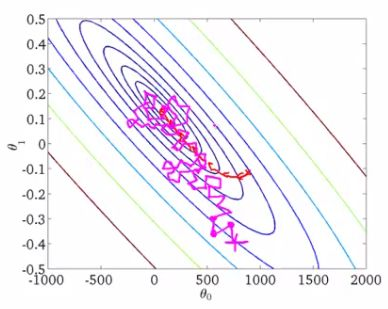评价：
优点：虽然SGD需要走很多步的样子，但是对梯度的要求很低（计算梯度快）。而对于引入噪声，大量的理论和实践工作证明，只要噪声不是特别大，SGD都能很好地收敛。应用大型数据集时，训练速度很快。比如每次从百万数据样本中，取几百个数据点，算一个SGD梯度，更新一下模型参数。相比于标准梯度下降法的遍历全部样本，每输入一个样本更新一次参数，要快得多。缺点：SGD在随机选择梯度的同时会引入噪声，使得权值更新的方向不一定正确。此外，SGD也没能单独克服局部最优解的问题。

动量优化法
NAG在Tensorflow中与Momentum合并在同一函数tf.train.MomentumOptimizer中，可以通过参数配置启用。

1. Momentum
使用动量(Momentum)的随机梯度下降法(SGD)，主要思想是引入一个积攒历史梯度信息动量来加速SGD。从训练集中取一个大小为

n

n

$n$的小批量{X(1),X(2),...,X(n)}$\left\{{X}^{\left(1\right)},{X}^{\left(2\right)},...,{X}^{\left(n\right)}\right\}$$\{X^{(1)}, X^{(2)},...,X^{(n)}\}$样本，对应的真实值分别为

Y(i)

Y

(

i

)

$Y^{(i)}$，则Momentum优化表达式为：

{vt=αvt−1+ηtΔJ(Wt,X(is),Y(is))Wt+1=Wt−vt

{

v

t

=

α

v

t

−

1

+

η

t

Δ

J

(

W

t

,

X

(

i

s

)

,

Y

(

i

s

)

)

W

t

+

1

=

W

t

−

v

t

\left\{ \begin{aligned} & v_t = \alpha v_{t-1} + \eta_t \Delta J(W_t, X^{(i_s)}, Y^{(i_s)}  ) \\ & W_{t+1} = W_t - v_t \end{aligned} \right.其中，

vt

v

t

$v_t$表示

t

t

$t$时刻积攒的加速度。α$\alpha$$\alpha$表示动力的大小，一般取值为0.9（表示最大速度10倍于SGD）。

ΔJ(Wt,X(is),Y(is))

Δ

J

(

W

t

,

X

(

i

s

)

,

Y

(

i

s

)

)

$\Delta J(W_t, X^{(i_s)}, Y^{(i_s)})$含义见SGD算法。

Wt

W

t

$W_t$表示

t

t

$t$时刻模型参数。
动量主要解决SGD的两个问题：一是随机梯度的方法（引入的噪声）；二是Hessian矩阵病态问题（可以理解为SGD在收敛过程中和正确梯度相比来回摆动比较大的问题）。
理解策略为：由于当前权值的改变会受到上一次权值改变的影响，类似于小球向下滚动的时候带上了惯性。这样可以加快小球向下滚动的速度。

2. NAG

牛顿加速梯度（NAG, Nesterov accelerated gradient）算法，是Momentum动量算法的变种。更新模型参数表达式如下：{vt=αvt−1+ηtΔJ(Wt−αvt−1)Wt+1=Wt−vt$\left\{\begin{array}{rl}& {v}_{t}=\alpha {v}_{t-1}+{\eta }_{t}\mathrm{\Delta }J\left({W}_{t}-\alpha {v}_{t-1}\right)\\ & {W}_{t+1}={W}_{t}-{v}_{t}\end{array}$\left\{ \begin{aligned} & v_t = \alpha v_{t-1} + \eta_t \Delta J(W_t - \alpha v_{t-1}  ) \\ &W_{t+1} = W_t - v_t \end{aligned} \right. 其中，

vt

v

t

$v_t$表示

t

t

$t$时刻积攒的加速度；α$\alpha$$\alpha$表示动力的大小；

ηt

η

t

$\eta_t$表示学习率；

Wt

W

t

$W_{t}$表示

t

t

$t$时刻的模型参数，ΔJ(Wt−αvt−1)$\mathrm{\Delta }J\left({W}_{t}-\alpha {v}_{t-1}\right)$$\Delta J(W_t - \alpha v_{t-1} )$表示代价函数关于

Wt

W

t

$W_t$的梯度。Nesterov动量梯度的计算在模型参数施加当前速度之后，因此可以理解为往标准动量中添加了一个校正因子。理解策略：在Momentun中小球会盲目地跟从下坡的梯度，容易发生错误。所以需要一个更聪明的小球，能提前知道它要去哪里，还要知道走到坡底的时候速度慢下来而不是又冲上另一个坡。计算

Wt−αvt−1

W

t

−

α

v

t

−

1

$W_t - \alpha v_{t-1}$可以表示小球下一个位置大概在哪里。从而可以提前知道下一个位置的梯度，然后使用到当前位置来更新参数。在凸批量梯度的情况下，Nesterov动量将额外误差收敛率从

O(1/k)(k步后)

O

(

1

/

k

)

(

k

步

后

)

$O(1/k)(k步后)$改进到

O(1/k2)

O

(

1

/

k

2

)

$O(1/k^2)$。然而，在随机梯度情况下，Nesterov动量对收敛率的作用却不是很大。

自适应学习率优化算法

思想：
算法描述：

Wt+1=Wt−η0∑tt′=1(gt′,i)+ϵ−−−−−−−−−−−−√⊙gt,i

W

t

+

1

=

W

t

−

η

0

∑

t

′

=

1

t

(

g

t

′

,

i

)

+

ϵ

⊙

g

t

,

i

W_{t+1}=W_t -\frac{\eta_0}{\sqrt{\sum_{t'=1}^t (g_{t',i})+\epsilon}} \odot g_{t,i}假定一个多分类问题，

i

i

$i$表示第i$i$$i$个分类，

t

t

$t$表示第t$t$$t$迭代同时也表示分类

i

i

$i$累计出现的次数。η0${\eta }_{0}$$\eta_0$表示初始的学习率取值一般为0.01，

ϵ

ϵ

$\epsilon$是一个取值很小的数（一般为1e-8）为了避免分母为0。

Wt

W

t

$W_t$表示

t

t

$t$时刻即第t$t$$t$迭代模型的参数，

gt,i=ΔJ(Wt,i)

g

t

,

i

=

Δ

J

(

W

t

,

i

)

$g_{t,i}=\Delta J(W_{t, i})$表示

t

t

$t$时刻，指定分类i$i$$i$，代价函数

J(⋅)

J

(

⋅

)

$J(\cdot)$关于

W

W

$W$的梯度。

2.RMSProp算法

思想：

算法描述：

RMSProp算法的一般策略可以表示为：⎧⎩⎨⎪⎪E[g2]t=αE[g2]t−1+(1−α)g2tWt+1=Wt−η0E[g2]t+ϵ−−−−−−−−√⊙gt$\left\{\begin{array}{rl}& E\left[{g}^{2}{\right]}_{t}=\alpha E\left[{g}^{2}{\right]}_{t-1}+\left(1-\alpha \right){g}_{t}^{2}\\ & {W}_{t+1}={W}_{t}-\frac{{\eta }_{0}}{\sqrt{E\left[{g}^{2}{\right]}_{t}+ϵ}}\odot {g}_{t}\end{array}$\left\{ \begin{aligned} & E[g^2]_t = \alpha  E[g^2]_{t-1} + (1-\alpha)g_t^2  \\ &W_{t+1} = W_t - \frac{\eta_0}{\sqrt{E[g^2]_t+\epsilon}} \odot g_t\end{aligned} \right. 其中，

Wt

W

t

$W_t$表示

t

t

$t$时刻即第t$t$$t$迭代模型的参数，

gt=ΔJ(Wt)

g

t

=

Δ

J

(

W

t

)

$g_t=\Delta J(W_t)$表示

t

t

$t$次迭代代价函数关于W$W$$W$的梯度大小，

E[g2]t

E

[

g

2

]

t

$E[g^2]_t$表示前

t

t

$t$次的梯度平方的均值。α$\alpha$$\alpha$表示动力（通常设置为0.9），

η0

η

0

$\eta_0$表示全局初始学习率。

ϵ

ϵ

$\epsilon$是一个取值很小的数（一般为1e-8）为了避免分母为0。RMSProp借鉴了Adagrad的思想，观察表达式，分母为

E[g2]t+ϵ−−−−−−−−√

E

[

g

2

]

t

+

ϵ

$\sqrt{E[g^2]_t+\epsilon}$。由于取了个加权平均，避免了学习率越来越低的的问题，而且能自适应地调节学习率。RMSProp算法在经验上已经被证明是一种有效且实用的深度神经网络优化算法。目前它是深度学习从业者经常采用的优化方法之一。

Δ

W

A

d

a

G

r

a

d

,

t

=

−

η

0

∑

t

′

=

1

t

(

g

t

′

,

i

)

+

ϵ

⊙

g

t

Δ

W

R

M

S

P

r

o

p

,

t

=

−

η

0

E

[

g

2

]

t

+

ϵ

⊙

g

t

\Delta W_{AdaGrad, \ t} = - \frac{\eta_0}{\sqrt{\sum_{t'=1}^t (g_{t',i})+\epsilon}} \odot g_t\\ \Delta W_{RMSProp, \ t} =- \frac{\eta_0}{\sqrt{E[g^2]_t+\epsilon}} \odot g_t

算法描述：

⎧⎩⎨⎪⎪⎪⎪⎪⎪⎪⎪⎪⎪E[g2]t=αE[g2]t−1+(1−α)g2tΔWt=−∑t−1i=1ΔWi−−−−−−−−−√E[g2]t+ϵ−−−−−−−−√Wt+1=Wt+ΔWt

{

E

[

g

2

]

t

=

α

E

[

g

2

]

t

−

1

+

(

1

−

α

)

g

t

2

Δ

W

t

=

−

∑

i

=

1

t

−

1

Δ

W

i

E

[

g

2

]

t

+

ϵ

W

t

+

1

=

W

t

+

Δ

W

t

\left\{ \begin{aligned} & E[g^2]_t = \alpha  E[g^2]_{t-1} + (1-\alpha)g_t^2  \\ & \Delta W_t = - \frac{\sqrt{\sum_{i=1}^{t-1}\Delta W_i }}{\sqrt{E[g^2]_t+\epsilon}}  \\ &W_{t+1} = W_t + \Delta W_t \end{aligned} \right.其中

Wt

W

t

$W_t$为第

t

t

$t$次迭代的模型参数，gt=ΔJ(Wt)${g}_{t}=\mathrm{\Delta }J\left({W}_{t}\right)$$g_t = \Delta J(W_t)$为代价函数关于

W

W

$W$的梯度。E[g2]t$E\left[{g}^{2}{\right]}_{t}$$E[g^2]_t$表示前

t

t

$t$次的梯度平方的均值。∑t−1i=1ΔWi$\sum _{i=1}^{t-1}\mathrm{\Delta }{W}_{i}$$\sum_{i=1}^{t-1}\Delta W_i$表示前

t−1

t

−

1

$t-1$次模型参数每次的更新步长累加求根。从表达式可以看出，AdaDelta不需要设置一个默认的全局学习率。
评价：

思想：
算法描述：

⎧⎩⎨⎪⎪⎪⎪⎪⎪⎪⎪⎪⎪⎪⎪⎪⎪⎪⎪mt=β1mt−1+(1−β1)gtvt=β2vt−1+(1−β2)g2tm^t=mt1−βt1,  v^t=vt1−βt2Wt+1=Wt−ηv^t−−√+ϵm^t

{

m

t

=

β

1

m

t

−

1

+

(

1

−

β

1

)

g

t

v

t

=

β

2

v

t

−

1

+

(

1

−

β

2

)

g

t

2

m

^

t

=

m

t

1

−

β

1

t

,

v

^

t

=

v

t

1

−

β

2

t

W

t

+

1

=

W

t

−

η

v

^

t

+

ϵ

m

^

t

\left\{ \begin{aligned} & m_t = \beta_1m_{t-1}+(1-\beta_1)g_t \\& v_t = \beta_2v_{t-1}+(1-\beta_2)g_t^2  \\ & \hat{m}_t = \frac{m_t}{1-\beta_1^t}, \ \  \hat{v}_t = \frac{v_t}{1-\beta_2^t} \\ & W_{t+1} = W_t -\frac{\eta}{\sqrt{\hat{v}_t}+\epsilon}\hat{m}_t\end{aligned} \right. 其中，

mt

m

t

$m_t$和

vt

v

t

$v_t$分别为一阶动量项和二阶动量项。

β1,β2

β

1

,

β

2

$\beta_1, \beta_2$为动力值大小通常分别取0.9和0.999；

m^t,v^t

m

^

t

,

v

^

t

$\hat{m}_t , \hat{v}_t$分别为各自的修正值。

Wt

W

t

$W_t$表示

t

t

$t$时刻即第t$t$$t$迭代模型的参数，

gt=ΔJ(Wt)

g

t

=

Δ

J

(

W

t

)

$g_t=\Delta J(W_t)$表示

t

t

$t$次迭代代价函数关于W$W$$W$的梯度大小；

ϵ

ϵ

$\epsilon$是一个取值很小的数（一般为1e-8）为了避免分母为0

各种优化器的比较
终于结束的漫长的理论分析，下面对各种优化器做一些有趣的比较。

1. 可视化比较
(1) 示例一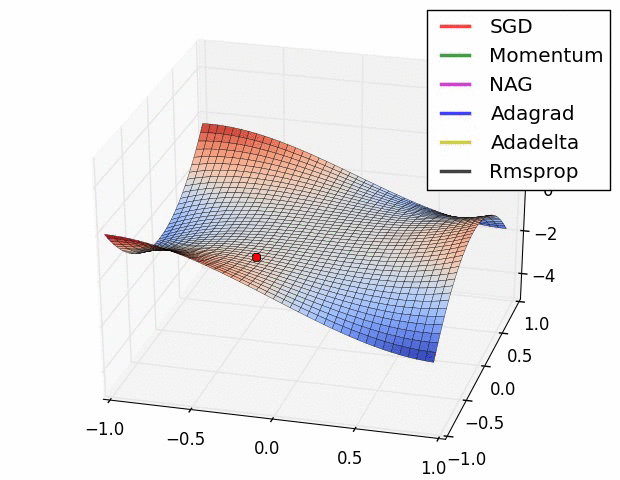上图描述了在一个曲面上，6种优化器的表现，从中可以大致看出：
① 下降速度：
② 下降轨迹：
SGD和三个自适应优化器轨迹大致相同。两个动量优化器初期走了“岔路”，后期也调整了过来。
(2) 示例二上图在一个存在鞍点的曲面，比较6中优化器的性能表现，从图中大致可以看出：
(3) 示例三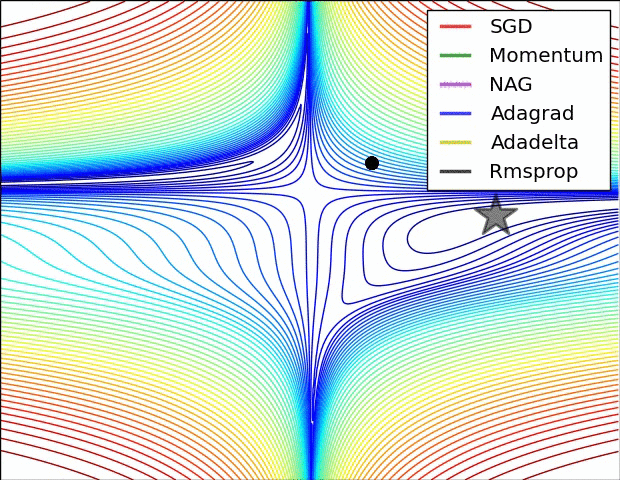上图比较了6种优化器收敛到目标点（五角星）的运行过程，从图中可以大致看出：
① 在运行速度方面
② 在收敛轨迹方面

2. 应用于简单分类问题的比较
Tensorflow中封装了一系列的优化器：
1. tf.train.GradientDescentOptimizer
5. tf.train.MomentumOptimizer
7. tf.train.FtrlOptimizer
10. tf.train.RMSPropOptimizer

下面采用选取几种优化器应用于UCI数据集iris.data简单的分类问题。为了简单起见，初始代码可以参考机器学习：过拟合、神经网络Dropout中没使用Dropout之前的代码。修改一行调用优化器的代码：
(1) 使用SGD优化器
优化器的代码为：
train_step = tf.train.GradientDescentOptimizer(0.2).minimize(loss)
运行结果
第1个训练周期训练集的准确率为：33.3%,  测试集的准确率为：32.2%
第2个训练周期训练集的准确率为：33.3%,  测试集的准确率为：32.2%
第3个训练周期训练集的准确率为：38.9%,  测试集的准确率为：35.6%
第4个训练周期训练集的准确率为：62.2%,  测试集的准确率为：62.7%
第5个训练周期训练集的准确率为：66.7%,  测试集的准确率为：66.1%
第6个训练周期训练集的准确率为：66.7%,  测试集的准确率为：67.8%
第7个训练周期训练集的准确率为：66.7%,  测试集的准确率为：67.8%
第8个训练周期训练集的准确率为：67.8%,  测试集的准确率为：67.8%
第9个训练周期训练集的准确率为：67.8%,  测试集的准确率为：67.8%
第10个训练周期训练集的准确率为：67.8%,  测试集的准确率为：67.8%

优化器的代码为：
train_step = tf.train.AdagradOptimizer(0.01).minimize(loss)
运行结果
第1个训练周期训练集的准确率为：33.3%,  测试集的准确率为：32.2%
第2个训练周期训练集的准确率为：35.6%,  测试集的准确率为：32.2%
第3个训练周期训练集的准确率为：66.7%,  测试集的准确率为：66.1%
第4个训练周期训练集的准确率为：66.7%,  测试集的准确率为：66.1%
第5个训练周期训练集的准确率为：66.7%,  测试集的准确率为：66.1%
第6个训练周期训练集的准确率为：66.7%,  测试集的准确率为：66.1%
第7个训练周期训练集的准确率为：66.7%,  测试集的准确率为：67.8%
第8个训练周期训练集的准确率为：66.7%,  测试集的准确率为：67.8%
第9个训练周期训练集的准确率为：67.8%,  测试集的准确率为：69.5%
第10个训练周期训练集的准确率为：68.9%,  测试集的准确率为：69.5%
(3) 使用Momentum优化器
优化器的代码为：
train_step = tf.train.MomentumOptimizer(learning_rate=0.01, momentum=0.9).minimize(loss)
运行结果
第1个训练周期训练集的准确率为：58.9%,  测试集的准确率为：61.0%
第2个训练周期训练集的准确率为：40.0%,  测试集的准确率为：42.4%
第3个训练周期训练集的准确率为：66.7%,  测试集的准确率为：66.1%
第4个训练周期训练集的准确率为：66.7%,  测试集的准确率为：66.1%
第5个训练周期训练集的准确率为：66.7%,  测试集的准确率为：66.1%
第6个训练周期训练集的准确率为：66.7%,  测试集的准确率为：66.1%
第7个训练周期训练集的准确率为：67.8%,  测试集的准确率为：67.8%
第8个训练周期训练集的准确率为：67.8%,  测试集的准确率为：67.8%
第9个训练周期训练集的准确率为：67.8%,  测试集的准确率为：69.5%
第10个训练周期训练集的准确率为：70.0%,  测试集的准确率为：67.8%

(4) 使用NAG优化器
优化器的代码为：
train_step = tf.train.MomentumOptimizer(learning_rate=0.01, momentum=0.9, use_nesterov=True).minimize(loss)
运行结果
第1个训练周期训练集的准确率为：37.8%,  测试集的准确率为：39.0%
第2个训练周期训练集的准确率为：66.7%,  测试集的准确率为：66.1%
第3个训练周期训练集的准确率为：66.7%,  测试集的准确率为：66.1%
第4个训练周期训练集的准确率为：66.7%,  测试集的准确率为：66.1%
第5个训练周期训练集的准确率为：66.7%,  测试集的准确率为：66.1%
第6个训练周期训练集的准确率为：66.7%,  测试集的准确率为：66.1%
第7个训练周期训练集的准确率为：66.7%,  测试集的准确率为：67.8%
第8个训练周期训练集的准确率为：67.8%,  测试集的准确率为：69.5%
第9个训练周期训练集的准确率为：70.0%,  测试集的准确率为：69.5%
第10个训练周期训练集的准确率为：71.1%,  测试集的准确率为：69.5%
点评：NAG和Momentum结果差不多，NAG似乎好那么一点点。
(5) 使用RMSProp优化器
优化器的代码为：
train_step = tf.train.RMSPropOptimizer(0.01).minimize(loss)
运行结果（RMSProp运行结果不稳定，下面是出现比较多的相近结果）
第1个训练周期训练集的准确率为：33.3%,  测试集的准确率为：32.2%
第2个训练周期训练集的准确率为：33.3%,  测试集的准确率为：32.2%
第3个训练周期训练集的准确率为：35.6%,  测试集的准确率为：35.6%
第4个训练周期训练集的准确率为：48.9%,  测试集的准确率为：49.2%
第5个训练周期训练集的准确率为：35.6%,  测试集的准确率为：35.6%
第6个训练周期训练集的准确率为：38.9%,  测试集的准确率为：42.4%
第7个训练周期训练集的准确率为：51.1%,  测试集的准确率为：50.8%
第8个训练周期训练集的准确率为：66.7%,  测试集的准确率为：66.1%
第9个训练周期训练集的准确率为：60.0%,  测试集的准确率为：62.7%
第10个训练周期训练集的准确率为：66.7%,  测试集的准确率为：66.1% 
点评：RMSProp优化器的效果有点不理想，而且不稳定。
优化器的代码为：
train_step = tf.train.AdamOptimizer(0.001).minimize(loss)
运行结果
第1个训练周期训练集的准确率为：33.3%,  测试集的准确率为：33.9%
第2个训练周期训练集的准确率为：36.7%,  测试集的准确率为：37.3%
第3个训练周期训练集的准确率为：66.7%,  测试集的准确率为：66.1%
第4个训练周期训练集的准确率为：66.7%,  测试集的准确率为：66.1%
第5个训练周期训练集的准确率为：66.7%,  测试集的准确率为：67.8%
第6个训练周期训练集的准确率为：67.8%,  测试集的准确率为：67.8%
第7个训练周期训练集的准确率为：67.8%,  测试集的准确率为：67.8%
第8个训练周期训练集的准确率为：71.1%,  测试集的准确率为：67.8%
第9个训练周期训练集的准确率为：71.1%,  测试集的准确率为：69.5%
第10个训练周期训练集的准确率为：71.1%,  测试集的准确率为：69.5%
train_step = tf.train.AdadeltaOptimizer(1).minimize(loss)
运行结果
第1个训练周期训练集的准确率为：60.0%,  测试集的准确率为：64.4%
第2个训练周期训练集的准确率为：66.7%,  测试集的准确率为：66.1%
第3个训练周期训练集的准确率为：66.7%,  测试集的准确率为：66.1%
第4个训练周期训练集的准确率为：66.7%,  测试集的准确率为：67.8%
第5个训练周期训练集的准确率为：67.8%,  测试集的准确率为：69.5%
第6个训练周期训练集的准确率为：70.0%,  测试集的准确率为：69.5%
第7个训练周期训练集的准确率为：72.2%,  测试集的准确率为：71.2%
第8个训练周期训练集的准确率为：73.3%,  测试集的准确率为：76.3%
第9个训练周期训练集的准确率为：75.6%,  测试集的准确率为：84.7%
第10个训练周期训练集的准确率为：76.7%,  测试集的准确率为：88.1%
总评：

优化器的选择

参考资料


展开全文机器学习 Optimizer Tensorflow
• ## Hive优化器

千次阅读 2018-03-12 23:20:12
1. 逻辑优化器LineageGenerato：各operator血缘情况的设定Columnpruner ：列前裁优化器Predicate PushDown：谓词下推优化器，将条件推到特定的位置Partition Pruner：分区减裁优化器PartitionCondition Pruner：消除...
1. 逻辑优化器
LineageGenerato：各operator血缘情况的设定
Columnpruner ：列前裁优化器
Predicate PushDown：谓词下推优化器，将条件推到特定的位置
Partition Pruner：分区减裁优化器
PartitionCondition Pruner：消除无用分支分区条件的优化器

Groupby optimizer：group优化map端预聚合的优化器
sampleprunner：抽样优化器，降低抽样的数量
Bucket map join：对分桶表做Mapjoin优化器
Sortedmergebucket map join：对sorted bucket表做map join 的优化
Unionprocessor：识别union两边的子查询是否都是map only的？
什么是map only呢？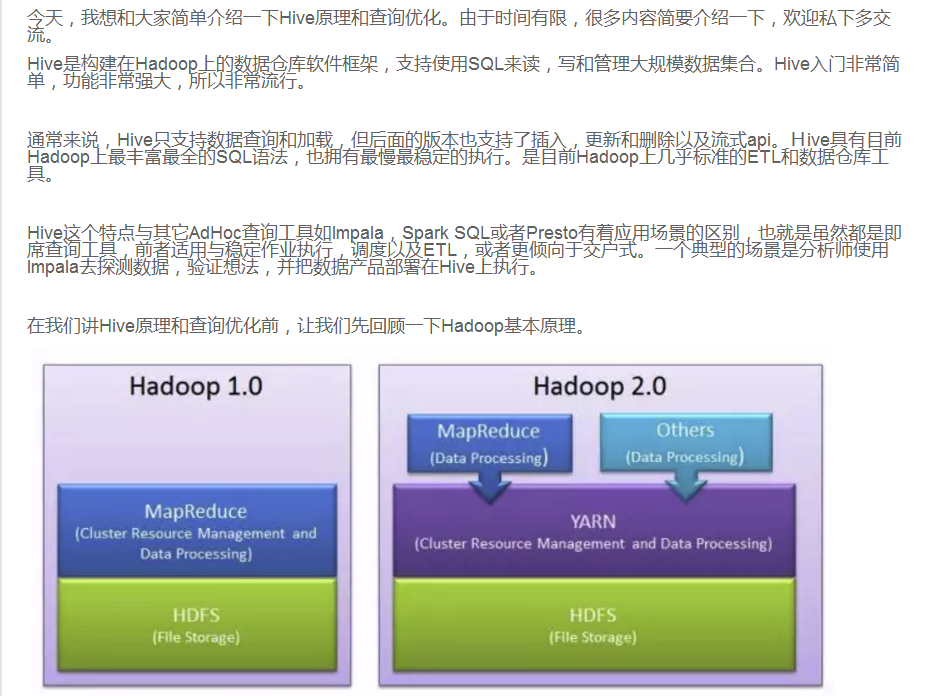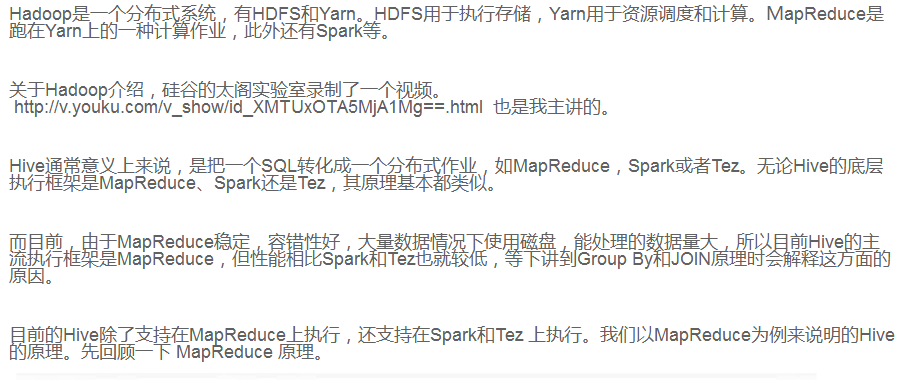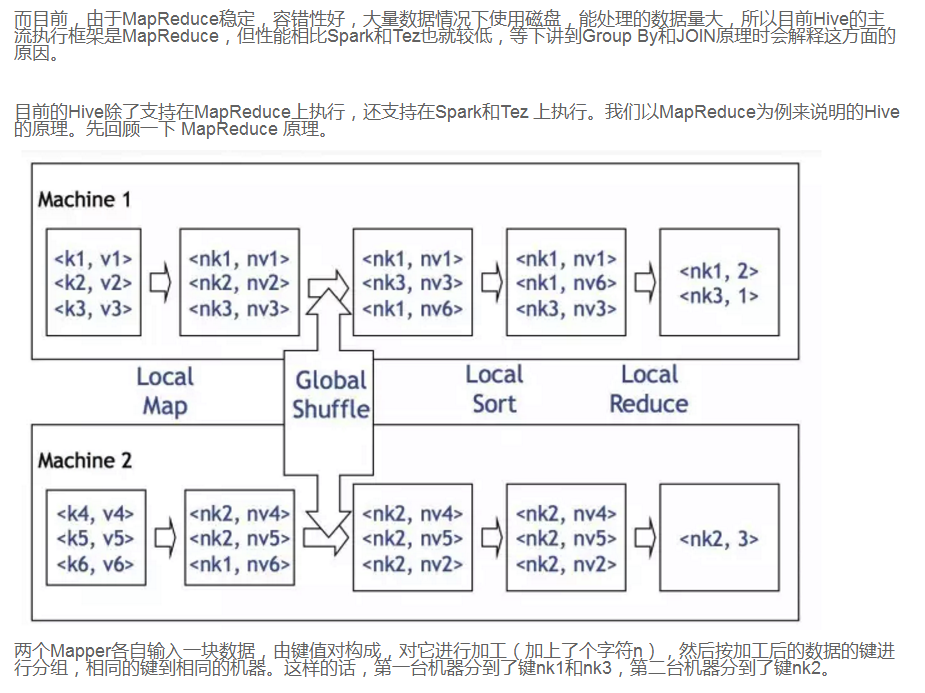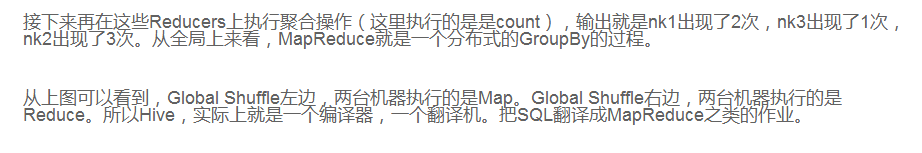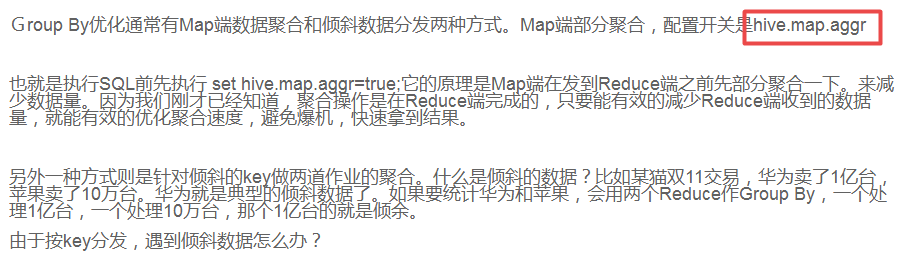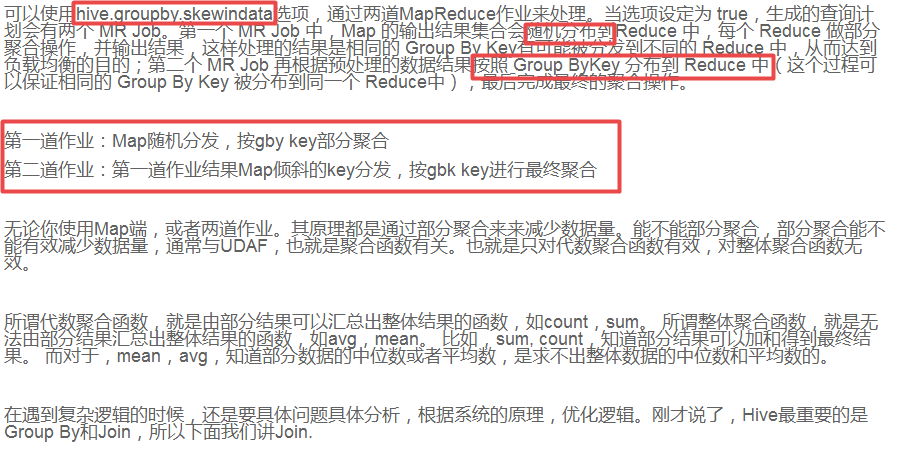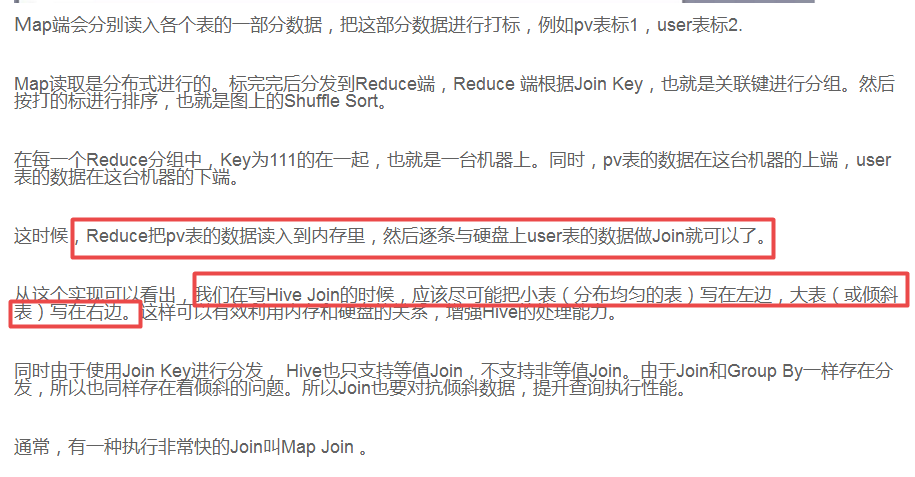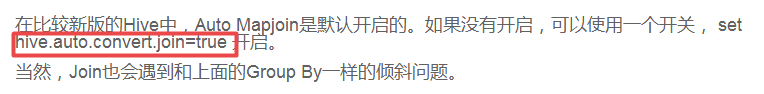展开全文Hive
• ## Pytorch优化器

千次阅读 2019-04-16 16:06:26
Pytorch优化器了解不同优化器构建一个优化器差别PyTorch种优化器选择 了解不同优化器 神经网络优化器，主要是为了优化我们的神经网络，使他在我们的训练过程中快起来，节省社交网络训练的时间。在pytorch中提供了...


Pytorch优化器
了解不同优化器构建一个优化器差别PyTorch种优化器选择

了解不同优化器
神经网络优化器，主要是为了优化我们的神经网络，使他在我们的训练过程中快起来，节省社交网络训练的时间。在pytorch中提供了torch.optim方法优化我们的神经网络，torch.optim是实现各种优化算法的包。最常用的方法都已经支持，接口很常规，所以以后也可以很容易地集成更复杂的方法。
构建一个优化器
要构造一个Optimizer，你必须给它一个包含参数（必须都是Variable对象）进行优化。然后，您可以指定optimizer的参 数选项，比如学习率，权重衰减等。具体参考torch.optim中文文档
optimizer = optim.SGD(model.parameters(), lr = 0.01, momentum=0.9)
optimizer = optim.Adam([var1, var2], lr = 0.0001)

差别

Stochastic Gradient Descent (SGD) SGD是最基础的优化方法，普通的训练方法, 需要重复不断的把整套数据放入神经网络NN中训练, 这样消耗的计算资源会很大.当我们使用SGD会把数据拆分后再分批不断放入 NN 中计算. 每次使用批数据, 虽然不能反映整体数据的情况, 不过却很大程度上加速了 NN 的训练过程, 而且也不会丢失太多准确率.

Momentum 传统的参数 W 的更新是把原始的 W 累加上一个负的学习率(learning rate) 乘以校正值 (dx). 此方法比较曲折。

RMSProp 有了 momentum 的惯性原则 , 加上 adagrad 的对错误方向的阻力, 我们就能合并成这样. 让 RMSProp同时具备他们两种方法的优势. 不过细心的同学们肯定看出来了, 似乎在 RMSProp 中少了些什么. 原来是我们还没把 Momentum合并完全, RMSProp 还缺少了 momentum 中的 这一部分. 所以, 我们在 Adam 方法中补上了这种想法.

Adam 计算m 时有 momentum 下坡的属性, 计算 v 时有 adagrad 阻力的属性, 然后再更新参数时 把 m 和 V 都考虑进去. 实验证明, 大多数时候, 使用 adam 都能又快又好的达到目标, 迅速收敛. 所以说, 在加速神经网络训练的时候, 一个下坡, 一双破鞋子, 功不可没.

# SGD 就是随机梯度下降
opt_SGD         = torch.optim.SGD(net_SGD.parameters(), lr=LR)
# momentum 动量加速,在SGD函数里指定momentum的值即可
opt_Momentum    = torch.optim.SGD(net_Momentum.parameters(), lr=LR, momentum=0.8)
# RMSprop 指定参数alpha
opt_RMSprop     = torch.optim.RMSprop(net_RMSprop.parameters(), lr=LR, alpha=0.9)

PyTorch种优化器选择
import torch
import torch.nn.functional as F
import matplotlib.pyplot as plt
import torch.utils.data as Data

# super param
LR = 0.01
BATCH_SIZE=32
EPOCH=12

x = torch.unsqueeze(torch.linspace(-1,1,1000),dim = 1)  #压缩为2维，因为torch 中 只会处理二维的数据
y = x.pow(2) + 0.2 * torch.rand(x.size())
print(x.numpy(),y.numpy())

torch_dataset = Data.TensorDataset(x, y)

dataset = torch_dataset,
batch_size = BATCH_SIZE,
shuffle = True,# true表示数据每次epoch是是打乱顺序抽样的
num_workers = 2, # 每次训练有两个线程进行的？？？？？ 改成 1 和 2 暂时没看出区别
)

class Net(torch.nn.Module):  # 继承 torch 的 Module
def __init__(self):
super(Net, self).__init__()     # 继承 __init__ 功能
# 定义每层用什么样的形式
self.hidden = torch.nn.Linear(1,20)   # 隐藏层线性输出
self.predict = torch.nn.Linear(20,1)   # 输出层线性输出

def forward(self, x):   # 这同时也是 Module 中的 forward 功能
# 正向传播输入值, 神经网络分析出输出值
x = F.relu(self.hidden(x))      # 激励函数(隐藏层的线性值)
x = self.predict(x)             # 输出值
return x

net_SGD = Net()
net_Momentum = Net()
net_RMSProp = Net()

opt_SGD = torch.optim.SGD(net_SGD.parameters(),lr=LR)
opt_Momentum = torch.optim.SGD(net_Momentum.parameters(),lr = LR,momentum=0.8) # 是SGD的改进，加了动量效果
opt_RMSProp = torch.optim.RMSprop(net_RMSProp.parameters(),lr=LR,alpha=0.9)
optimizers = [opt_SGD, opt_Momentum, opt_RMSProp, opt_Adam]
# 比较这4个优化器会发现，并不一定越高级的效率越高，需要自己找适合自己数据的优化器

loss_func = torch.nn.MSELoss()
losses_his = [[],[],[],[]]

if __name__ == '__main__': # EPOCH + win10 需要if main函数才能正确运行，
for epoch in range(EPOCH):
print(epoch)
b_x = Variable(batch_x)
b_y = Variable(batch_y)

for net,opt,l_his in zip(nets, optimizers, losses_his):
output = net(b_x)  # get_out for every net
loss = loss_func(output,b_y)  # compute loss for every net
loss.backward()
l_his.append(loss.data) # loss recoder

for i,l_his in enumerate(losses_his):
plt.plot(l_his,label=labels[i])
plt.legend(loc='best')
plt.xlabel('Steps')
plt.ylabel('Loss')
plt.ylim = ((0,0.2))
plt.show()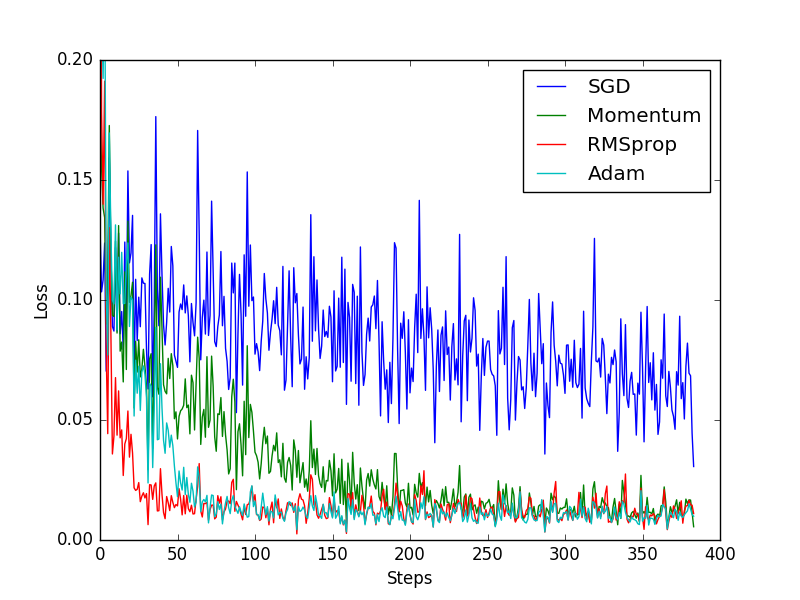SGD 是最普通的优化器, 也可以说没有加速效果, 而 Momentum 是 SGD 的改良版, 它加入了动量原则. 后面的 RMSprop 又是 Momentum 的升级版. 而 Adam 又是 RMSprop 的升级版. 不过从这个结果中我们看到, Adam 的效果似乎比 RMSprop 要差一点. 所以说并不是越先进的优化器, 结果越佳. 我们在自己的试验中可以尝试不同的优化器, 找到那个最适合你数据/网络的优化器.
参考 https://morvanzhou.github.io/tutorials/machine-learning/torch/3-06-optimizer/ https://ptorch.com/news/54.html
展开全文• 不过MySQL查询优化器只对少部分查询不适用，而且我们往往可以通过改写查询让MySQL高效的完成工作。 1 关联子查询 MySQL的子查询实现的非常糟糕。最糟糕的一类查询时where条件中包含in()的子查询语句。因为MySQL对...
MySQL的万能嵌套循环并不是对每种查询都是最优的。不过MySQL查询优化器只对少部分查询不适用，而且我们往往可以通过改写查询让MySQL高效的完成工作。
1 关联子查询
MySQL的子查询实现的非常糟糕。最糟糕的一类查询时where条件中包含in()的子查询语句。因为MySQL对in()列表中的选项有专门的优化策略，一般会认为MySQL会先执行子查询返回所有in()子句中查询的值。一般来说，in()列表查询速度很快，所以我们会以为sql会这样执行
select * from tast_user where id in (select id from user where name like '王%');
我们以为这个sql会解析成下面的形式
select * from tast_user where id in (1,2,3,4,5);
实际上MySQL是这样解析的
select * from tast_user where exists
(select id from user where name like '王%' and tast_user.id = user.id);
MySQL会将相关的外层表压缩到子查询中，它认为这样可以更高效的查找到数据行。
这时候由于子查询用到了外部表中的id字段所以子查询无法先执行。通过explin可以看到，MySQL先选择对tast_user表进行全表扫描，然后根据返回的id逐个执行子查询。如果外层是一个很大的表，那么这个查询的性能会非常糟糕。当然我们可以优化这个表的写法：
select tast_user.* from tast_user inner join user using(tast_user.id) where user.name like '王%'
另一个优化的办法就是使用group_concat()在in中构造一个由逗号分隔的列表。有时这比上面使用关联改写更快。因为使用in()加子查询，性能通常会非常糟糕。所以通常建议使用exists()等效的改写查询来获取更好的效率。
如何书写更好的子查询就不在介绍了，因为现在基本都要求拆分成单表查询了，有兴趣的话可以自行去了解下。
2 UNION的限制
有时，MySQL无法将限制条件从外层下推导内层，这使得原本能够限制部分返回结果的条件无法应用到内层查询的优化上。
如果希望union的各个子句能够根据limit只取部分结果集，或者希望能够先排好序在合并结果集的话，就需要在union的各个子句中分别使用这些子句。例如，想将两个子查询结果联合起来，然后在取前20条，那么MySQL会将两个表都存放到一个临时表中，然后在去除前20行。
(select first_name,last_name from actor order by last_name) union all
(select first_name,last_name from customer order by  last_name) limit 20;
这条查询会将actor中的记录和customer表中的记录全部取出来放在一个临时表中，然后在取前20条，可以通过在两个子查询中分别加上一个limit 20来减少临时表中的数据。
现在中间的临时表只会包含40条记录了，处于性能考虑之外，这里还需要注意一点：从临时表中取出数据的顺序并不是一定，所以如果想获得正确的顺序，还需要在加上一个全局的order by操作
3 索引合并优化
前面文章中已经提到过，MySQL能够访问单个表的多个索引以合并和交叉过滤的方式来定位需要查找的行。
4 等值传递
某些时候，等值传递会带来一些意想不到的额外消耗。例如，有一个非常大的in()列表，而MySQL优化器发现存在where/on或using的子句，将这个列表的值和另一个表的某个列相关联。
那么优化器会将in()列表都赋值应用到关联的各个表中。通常，因为各个表新增了过滤条件，优化器可以更高效的从存储引擎过滤记录。但是如果这个列表非常大，则会导致优化和执行都会变慢。
5 并行执行
MySQL无法利用多核特性来并行执行查询。很多其他的关系型数据库鞥能够提供这个特性，但MySQL做不到。这里特别指出是想提醒大家不要花时间去尝试寻找并行执行查询的方法。
6 哈希关联
7 松散索引扫描
由于历史原因，MySQL并不支持松散索引扫描，也就无法按照不连续的方式扫描一个索引。通常，MySQL的索引扫描需要先定义一个起点和重点，即使需要的数据只是这段索引中很少的几个，MySQL仍需要扫描这段索引中每个条目。
例：现有索引（a,b）
select * from table where b between 2 and 3;
因为索引的前导字段是a，但是在查询中只指定了字段b，MySQL无法使用这个索引，从而只能通过全表扫描找到匹配的行。
MySQL全表扫描：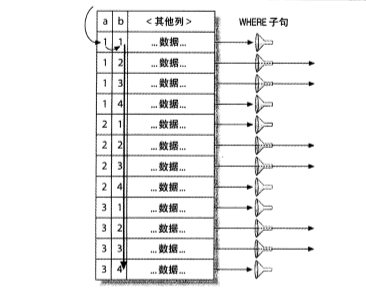了解索引的物理结构的话，不难发现还可以有一个更快的办法执行上面的查询。索引的物理结构不是存储引擎的API使得可以先扫描a列第一个值对应的b列的范围，然后在跳到a列第二个不同值扫描对应的b列的范围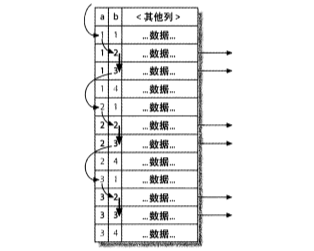这时就无需在使用where子句过滤，因为松散索引扫描已经跳过了所有不需要的记录。
上面是一个简单的例子，处理松散索引扫描，新增一个合适的索引当然也可以优化上述查询。但对于某些场景，增加索引是没用的，例如，对于第一个索引列是范围条件，第二个索引列是等值提交建查询，靠增加索引就无法解决问题。
MySQL5.6之后，关于松散索引扫描的一些限制将会通过索引条件吓退的分行是解决。
8 最大值和最小值优化
对于MIN()和MAX()查询，MySQL的优化做的并不好，例：
select min(actor_id) from actor where first_name = 'wang'
因为在first_name字段上并没有索引，因此MySQL将会进行一次全表扫描。如果MySQL能够进行主键扫描，那么理论上，当MySQL读到第一个太满足条件的记录的时候就是我们需要的最小值了，因为主键是严哥按照actor_id字段的大小排序的。但是MySSQL这时只会做全表扫描，我们可以通过show status的全表扫描计数器来验证这一点。一个区县优化办法就是移除min()函数，然后使用limit 1来查询。
这个策略可以让MySQL扫描尽可能少的记录数。这个例子告诉我们有时候为了获得更高的性能，就得放弃一些原则。
9 在同一个表上查询和更新
MySQL不允许对同一张表同时进行查询和更新。这并不是优化器的限制，如果清楚MySQL是如何执行查询的，就可以避免这种情况。例：
update table set cnt = (select count(*) from table as tb where tb.type = table.type);

这个sql虽然符合标准单无法执行，我们可以通过使用生成表的形式绕过上面的限制，因为MySQL只会把这个表当做一个临时表来处理。
update table inner join
(select type,count(*) as cnt from table group by type) as tb using(type)
set table.cnt = tb.cnt;
实际上这执行了两个查询：一个是子查询中的select语句，另一个是夺标关联update，只是关联的表时一个临时表。子查询会在update语句打开表之前就完成，所以会正常执行。
10 查询优化器的提示（hint）
如果对优化器选择的执行计划不满意，可以使用优化器提供的几个提示（hint）来控制最终的执行计划。下面将列举一些常见的提示，并简单的给出什么时候使用该提示。通过在查询中加入响应的提示，就可以控制该查询的执行计划。
① HIGH_PRIORITY 和 LOW_PRIORITY
这个提示告诉MySQL，当多个语句同时访问某一表的时候，哪些语句的优先级相对高些，哪些语句优先级相对低些。
HIGH_PRIORITY用于select语句的时候，MySQL会将此select语句重新调度到所有正在表锁以便修改数据的语句之前。实际上MySQL是将其放在表的队列的最前面，而不是按照常规顺序等待。HIGH_PRIORITY还可以用于insert语句，其效果只是简单的体校了全局LOW_PRIORITY设置对该语句的影响。
LOW_PRIORITY则正好相反，它会让语句一直处于等待状态，只要在队列中有对同一表的访问，就会一直在队尾等待。在CRUD语句中都可以使用。
这两个提示只对使用表锁的存储引擎有效，不能在InnoDB或其他有细粒度所机制和并发控制的引擎中使用。在MyISAM中也要慎用，因为这两个提示会导致并发插入被禁用，可能会严重降低性能。
HIGH_PRIORITY和LOW_PRIORITY其实只是简单的控制了MySQL访问某个数据表的队列顺序。
② DELAYED
这个提示对insert和replace有效。MySSQL会将使用该提示的语句立即返回给客户端，并将插入的行数据放入缓冲区，然后在表空闲时批量将数据写入。日志型系统使用这样的提示非常有效，或者是其他需要写入大量数据但是客户端却不需要等待单条语句完成I/O的应用。这个用法有一些限制。并不是所有的存储引擎都支持，并且该提示会导致函数last_insert_id()无法正常工作。
③ STRAIGHT_JOIN
这个提示可以防止在select语句的select关键字之后，也可以防止在任何两个关联表的名字之间。第一个用法是让查询中所有的表按照在语句中出现的顺序进行关联。第二个用法则是固定其前后两个表的关联顺序。
当MySQL没能选择正确的关联顺序的时候，或者由于可能的顺序太多导致MySQL无法评估所有的关联顺序的时候，STRAIGHT_JOIN都会很有用，在MySQL可能会发给大量时间在statistics状态时，加上这个提示则会大大减少优化器的搜索空间
④ SQL_SMALLRESULT和SQL_BIG_RESULT
这个两个提示只对select语句有效。他们告诉优化器对group by或者distinct查询如何使用临时表及排序。SQL_SMALL_RESULT告诉优化器结果集会很小，可以将结果集放在内存中的索引临时表，以避免排序操作。如果是SQL_BIG_RESULT，则会告诉优化器结果集可能会非常大，建议使用磁盘临时表做排序操作。
⑤ SQL_BUFFER_RESULT
这个提示告诉优化器将查询结果放入一个临时表，然后尽可能快速释放表锁。这和前面提到的由客户端缓存结果不同。当你无法使用客户端缓存的时候，使用服务器端的缓存通常很有效。好处是无需在客户端上消耗过多内存，还能尽快释放表锁。代价是服务器端将需要更多的内存。
⑥ SQL_CACHE和SQL_NO_CACHE
这个提示告诉MySQL这个结果集是否应该放入查询缓存中。
⑦ SQL_CALC_FOUND_ROWS
严哥来说，这并不是一个优化器提示。它不会告诉优化器任何关于执行计划的东西。它会让MySQL返回的结果集包含更多的信息。查询中加上该提示MySQL会计算limit子句之后这个查询要返回的结果集总数，而实际上值返回limit要求的结果集。可以通过函数found_row()获得这个值。慎用，后面会说明为什么。
⑧ FOR UPDATE和LOCK IN SHARE MODE
这也不是真正的优化器提示。这两个提示主要控制select语句的锁机制，但只对实现了行级锁的存储引擎有效。使用该提示会对符合查询条件的数据行加锁。对于insert/select语句是不需要这两个提示的因为5.0以后会默认给这些记录加上读锁。
唯一内置的支持这两个提示的引擎就是InnoDB，可以禁用该默认行为。另外需要记住的是，这两个提示会让某些优化无法正常使用，例如索引覆盖扫描。InnoDB不能在不访问主键的情况下排他的锁定行，因为行的版本信息保存在主键中。
如果这两个提示被经常滥用，很容易早晨服务器的锁争用问题。
⑨ USE INDEX、IGNORE INDEX和FORCE INDEX
这几个提示会告诉优化器使用或者不使用那些索引来查询记录。
在5.0版本以后新增了一些参数来控制优化器的行为：
① optimizer_search_depth
这个参数控制优化器在穷举执行计划时的限度。如果查询长时间处于statistics状态，那么可以考虑调低此参数。
② optimizer_prune_level
该参数默认是打开的，这让优化器会根据需要扫描的行数来决定是否跳过某些执行计划。
③optimizer_switch
这个变量包含了一些开启/关闭优化器特性的标志位。
前面两个参数时用来控制优化器可以走的一些捷径。这些捷径可以让优化器在处理非常复杂的SQL语句时，可以更高效，但也可能让优化器错过一些真正最优的执行计划，所以慎用。
修改优化器提示可能在MySQL更新后让新版的优化策略失效，所以一定要谨慎。
展开全文MySQL优化 查询性能优化
• tensorflow详解优化器的选择1. 什么是优化器2. 梯度下降算法 1. 什么是优化器 要型对各种优化的选择游刃有余，得先理解什么是优化器。 深度学习的目标是通过不断改变网络参数，使得参数能够对输入做各种非线性...梯度下降优化

万次阅读 多人点赞 2020-09-13 15:26:30深度学习 adam算法
• ## mysql查询优化器

千次阅读 2019-03-15 00:55:44
优化器分类 传统关系型数据库里面的优化器分为CBO和RBO两种。 RBO— Rule_Based Potimizer 基于规则的优化器: RBO :RBO所用的判断规则是一组内置的规则，这些规则是硬编码在数据库的编码中的，RBO会根据这些规则...

万次阅读 多人点赞 2018-06-22 12:34:24
• MySQL 优化器使用基于成本的优化方式（Cost-based Optimization），利用内置的成本模型和数据字典信息以及存储引擎的统计信息决定使用哪些步骤实现查询语句，也就是查询计划。同时，MySQL 为我们提供了控制优化器的...mysql 直方图统计

千次阅读 2020-07-31 21:54:32机器学习 深度学习 人工智能 python 算法
• 平衡优化器（EO）是一种新颖的优化算法，受控制体积质量平衡的启发，可以估算动态状态和平衡状态。在EO中，搜索代理会针对某些称为平衡候选物的有才干的粒子随机更新其浓度（位置），以最终达到平衡状态（最佳结果）...
• ## pytorch优化器详解：SGD

万次阅读 多人点赞 2020-08-31 10:07:01
通常偏导数不会直接作用到对应的参数p上，而是通过优化器做一下处理，得到一个新的值，计算过程用函数F表示（不同的优化器对应的F的内容不同），即，然后和学习率lr一起作用于可学习参数p，即。 SGD参数 SGD是随机...深度学习 python 随机梯度下降
• ## Oracle优化器

千次阅读 2015-12-21 15:46:19
Oracle优化器三种： 第一种： RBO优化器（基于规则） a. RULE(基于规则) b. COST (基于成本) c. CHOOSE (选择性) 是一种基于规则的优化器 a:也是RBO全称（Cost-Based Optimizer）随着CBO是基于成本的...
• 2020智能优化算法：平衡优化器算法 文章目录2020智能优化算法：平衡优化器算法1.算法背景2.算法优化原理3.实验结果4.参考文献5.Matlab代码 摘要：平衡优化器（equilibrium optimizer, EO）是于2020年提出的一种全新...算法 机器学习 人工智能 python
• 这一次借着 TiDB 优化器重构的契机，我们将这个系列再向着数据库的核心前进一步，挑战一下「为 TiDB 的优化器增加优化规则」，带大家初步体验一下可以对查询的执行时间产生数量级影响的优化器的魅力。 众所周知优化...TiDB 数据库 开源社区
• ## pytorch优化器详解：RMSProp

万次阅读 多人点赞 2020-09-10 18:19:24
通常偏导数不会直接作用到对应的可学习参数p上，而是通过优化器做一下处理，得到一个新的值，处理过程用函数F表示（不同的优化器对应的F的内容不同），即，然后和学习率lr一起用于更新可学习参数p，即。 RMSProp...深度学习 pytorch rmsprop
• ## Tensorflow 优化器（optimizer）

万次阅读 多人点赞 2018-12-11 19:06:10
所谓的优化器，就是tensorflow中梯度下降的策略，用于更新神经网络中数以百万的参数。工程师们除了在不断的推出新的神经网络的结构以外，还在不断的推出新的参数更新的策略，在这篇博客中，我们就列举tensorflow中...梯度下降 参数学习 tensorflow
• 查询优化器的基本原理小明考上了北清大学的计算机研究生，今年学校开了数据库原理的课程，小明对查询优化的内容不是很理解，虽然已经使出了洪荒之力，仍觉得部分原理有些晦涩难懂，于是打算问一下自己的哥哥大明。...postgresql
• 这是一个实现实现梯度下降算法的优化器类，用于构造一个新的梯度下降优化器实例   构造器方法__init__ __init__( learning_rate, use_locking = False, name = ' GradientDescent ' ) 参数: learnin...
• Oracle优化器的优化方式和优化模式 Oracle在执行一个SQL之前,首先要分析一下语句的执行计划,然后再按执行计划去执行。分析语句的执行计划的工作是由优化器(Optimizer) 来完成的。不同的情况,一条SQL可能有多种执行...
• [MCGC] Grand Theft Auto V 网络优化器写在前面■■■ 使用说明 ■■■[错误]该目录没有资料库文件，或资料库文件被占用■■■ 异常情况须知 ■■■ 写在前面 软件版本：2.0.1.19 公测版 若网络是移动铁通，优化...GTA5
• ## 如何选择优化器 optimizer

万次阅读 多人点赞 2017-06-14 12:17:01机器学习 深度学习
• 文章目录torch.optim 优化器使用torch.optim:进行单次优化 optimizer.step()使用闭包优化 optimizer.step(closure)常用的优化器函数学习率 与学习率调节 torch.optim 优化器 使用torch.optim: 构建一个...
• 搭建模型时，可以选择收敛速度最快的优化器，以提升训练速度；输出结果时，建议每个优化器都尝试一遍，最后选择准确率最高的优化器。没有哪个优化器绝对好，只有尝试了才知道。 import tensorflow as tf from ...tensorflow 深度学习
• 基于Sigmund的经典99行代码，将其中的OC优化器改成MMA优化器，包含所需变量定义和函数调用
• 文章目录一、Oracle的优化器1.1 优化器简介1.2 SQL执行过程二、优化器优化方式2.1 优化器的优化方式2.2 基于规则的优化器2.2.1 RBO简介2.2.2 RBO缺陷2.2.3 RBO执行过程2.2.4 RBO特殊情况2.2.5 强制CBO的情况2.3 基于...Oracle
• 手册上MYSQL查询优化器概述；个人对MySQL优化器的理解；分析优化器优化过程中的信息；调节MySQL优化器的优化等mysql 优化 性能
• 综述：神经网络的优化-优化器，动量，自适应学习率，批量归一化 xingbod@gmail.com 在本文中，我将讨论与神经网络优化有关的以下概念： 优化面临的挑战 动量 适应性学习率 参数初始化 批量归一化 优化面临的挑战 ...神经网络 深度学习 机器学习 大数据 算法
• 训练神经网络的时候不是应该用BP算法吗，为什么tensorflow中用梯度下降算法的优化器 就能实现对于神经网络权值的更新？tensorflow
• 优化器 optimzier优化器的作用：优化器就是需要根据网络反向传播的梯度信息来更新网络的参数，以起到降低loss函数计算值的作用。 从优化器的作用出发，要使得优化器能够起作用，需要主要两个东西： 优化器需要知道...pytorch...